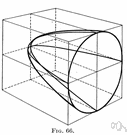paraboloid

(redirected from Elliptic paraboloid)
Also found in: Thesaurus, Encyclopedia.

pa·rab·o·loid

(pə-răb′ə-loid′)
n.
A surface having parabolic sections parallel to a single coordinate axis and elliptic or circular sections perpendicular to that axis.

paraboloid

(pəˈræbəˌlɔɪd)
n
(Mathematics) a geometric surface whose sections parallel to two coordinate planes are parabolic and whose sections parallel to the third plane are either elliptical or hyperbolic. Equations x2/a2 ± y2/b2 = 2cz
Collins English Dictionary – Complete and Unabridged, 12th Edition 2014 © HarperCollins Publishers 1991, 1994, 1998, 2000, 2003, 2006, 2007, 2009, 2011, 2014

pa•rab•o•loid

(pəˈræb əˌlɔɪd)

n.
a surface that can be put into a position such that its sections parallel to at least one coordinate plane are parabolas.
[1650–60]
 Noun 1paraboloid - a surface having parabolic sections parallel to a single coordinate axis and elliptic sections perpendicular to that axisplane figure, two-dimensional figure - a two-dimensional shape